# A variety of graphics that express "concept of mathematics" visually and beautifully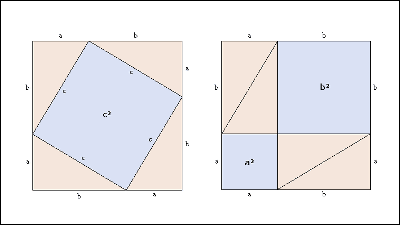The "beauty of mathematics" is said to be the first time to gain by understanding mathematics deeply. Mathematics dislikes may become a little better when beauty is transmitted, but mathematicians who are eager to think about their teeth because many people who dislike mathematics can not convey beauty in the first place in the first place . The graphics collection that can visually understand the concept of mathematics convenient at such time is as follows.

Soft question - Visually stunning math concepts which are easy to explain - Mathematics Stack Exchange
http://math.stackexchange.com/questions/733754/visually-stunning-math-concepts-which-are-easy-to-explain

01: sum of odd numbers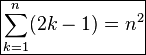The sum of odd numbersSquare numberIt will be obvious if you look at the figure below to become.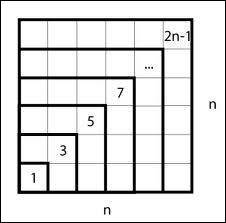02: Even number sum

It is easy to understand that the sum of even numbers becomes n × (n + 1).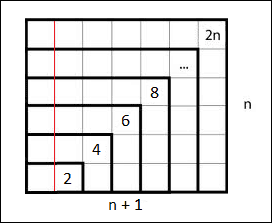03: Deployment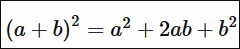Expansion of quadratic equations can be illustrated like this.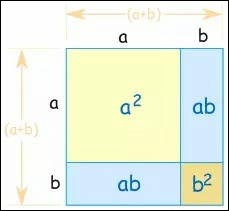04: Sum of outer angles of polygons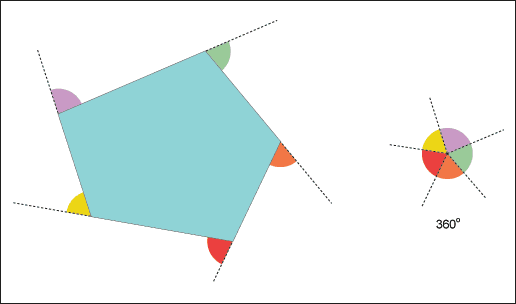The sum of the outer angles of polygons is 360 degrees, you can intuitively understand such an animation.05: Area of ​​diamond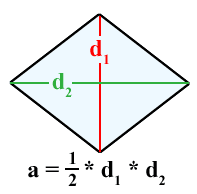The area of ​​the diamond is rather confusing ... ...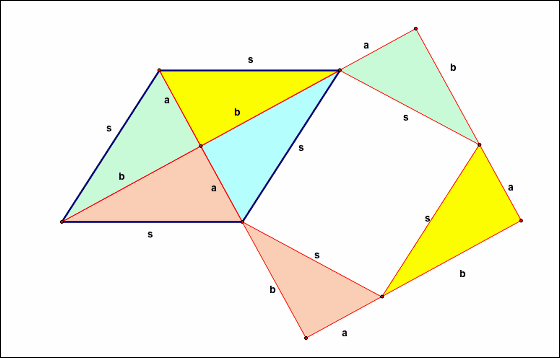Also the area of ​​diamonds. This is what you think about dividing the diamond into two triangles, rather than a visual understanding rather than an idea of ​​the calculation method.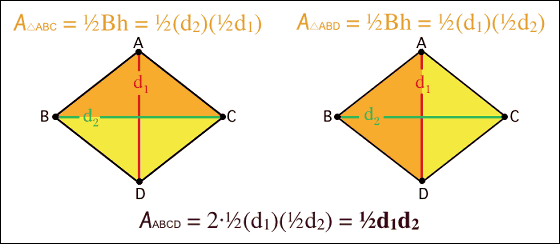06: Pythagorean theorem (Theorem of Sanchigata)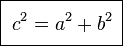Pythagorean theorem (Sanpei no Theorem)I can prove with this feeling.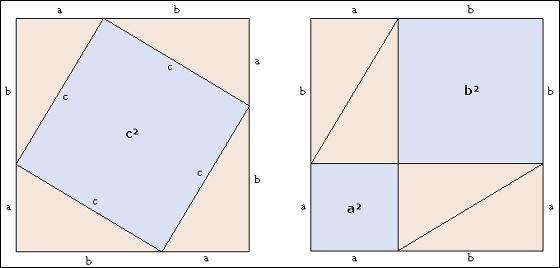This figure proved using the circle and the right triangle the theorem of the Triple. Pythagorean theorem will appear when transforming the relational expression obtained from the figure.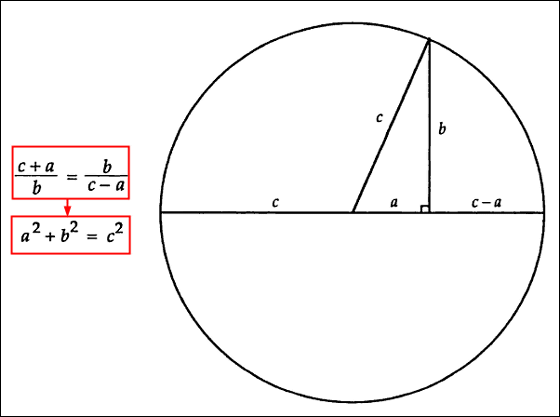The figure giving the proof of the most common theorem of Mikiga is this. Very simple and clear.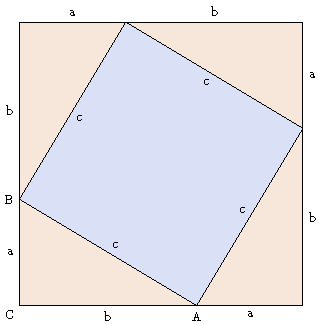It is such feeling when doing demonstration experiment of Sanpei's theorem.

Pythagorean theorem water demo - YouTube

07: Sum of infinite equi ratio series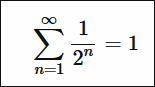1/2 + 1/4 + 1/8 + ...... If you add infinitely, you can express it as "1" in the figure below.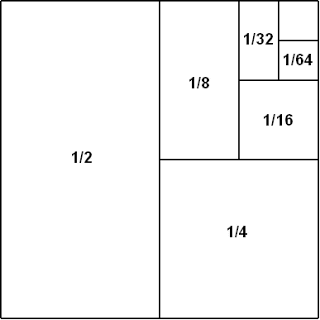The sum of the infinite series of 1/2 + 1/4 + 1/8 + ..... can also be expressed using triangles.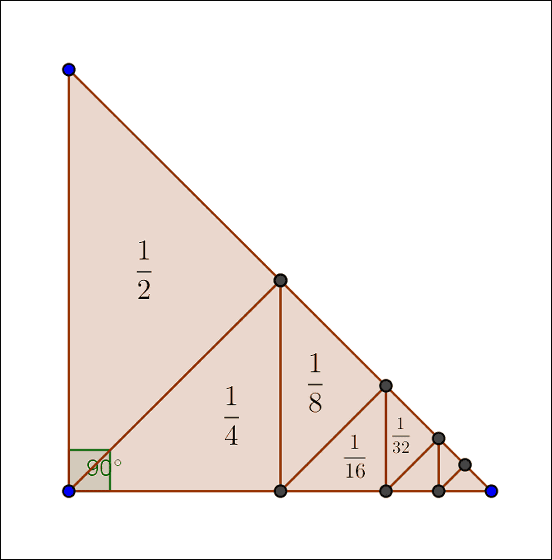08: Pi

I can understand about the circumference ratio (π) about 3.14 by looking at the animation below.09: Area of ​​circle

Why the area of ​​the circle is radius × radius × π is like this. Cut the circles very finely and rearrange them to get closer to a rectangle.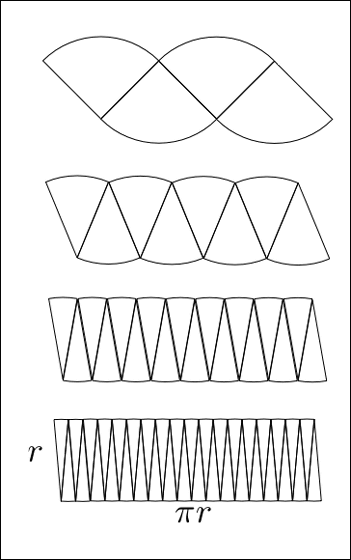10: Golden ratio

Golden ratioHow to draw.11: sine (sign) · cosine (cosine)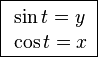You can understand why the graph of sine / cosine is a waveform and whether the phase is π / 2 rad (radian) different, if it is the graphic below.12: Circularity method

Indicate the angle of the international unit systemRadiansFig.13: Taylor expansion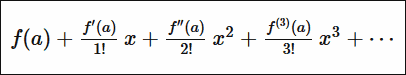Taylor expansionIt is a schematic diagram of such feeling.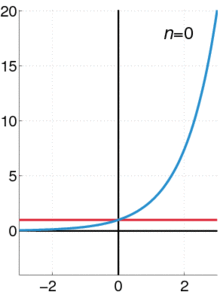14: Logarithmic helix

Logarithmic spiralThe trajectory of the fixed point when rolling is like this. Surprisingly it seems that the origin part draws a straight line.15: Fourier transform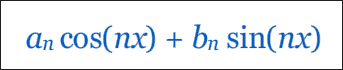Fourier transformWhen visualizing it, it looks like this.The Fourier transform can also be expressed as below.16: Line integral

Along the smooth curve of the scalar fieldLine integralIt is like this.17: Multiplication table

This is a diagram in which figures are arranged according to a certain rule called a multiplication table. A beautiful pattern is drawn by changing the color from 1 to a specified number (modulo) from blue to red. Modulo = 15 is like this.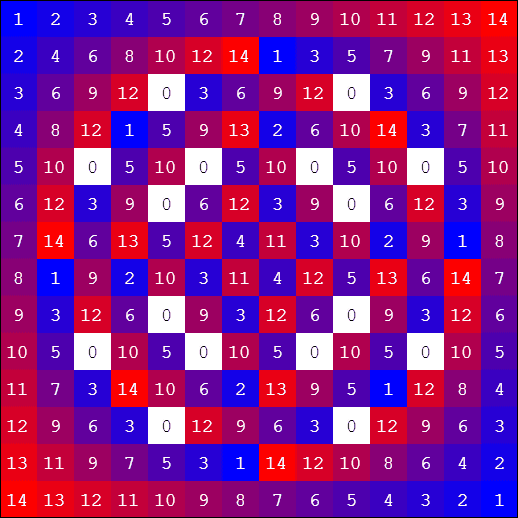When modulo = 512, it seems that there is a gradation.This siteYou can create a pattern with.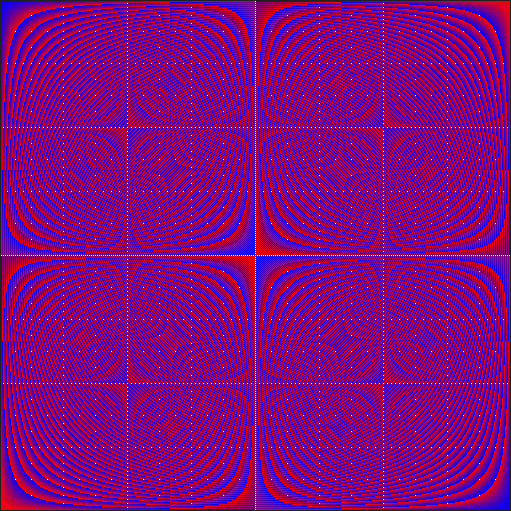18: Mandelbrot assembly

Mandelbrot setWas usedFractal designExamples.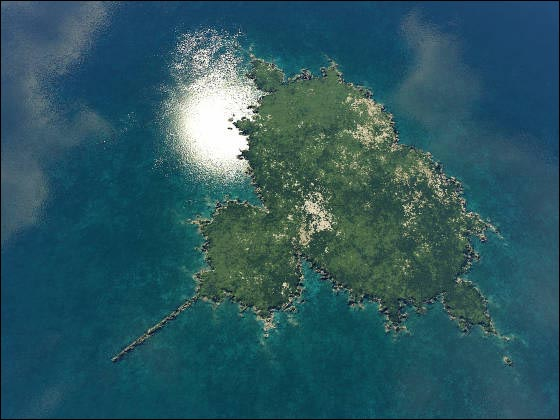The whole shape and part of the figureSelf-similarityIt becomes such a mysterious shape when it becomes.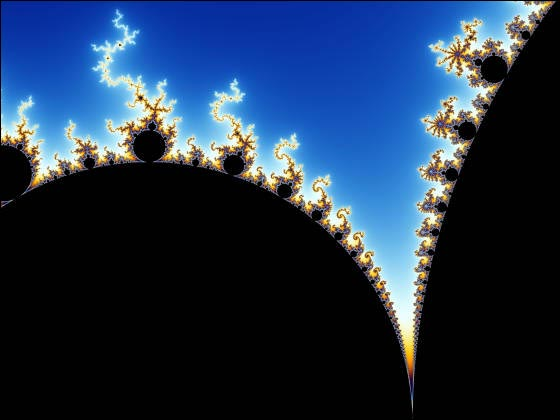19: Pendulum and magnet

A diagram in which the three magnets of red, blue, and yellow are plotted on the horizontal plane so as to draw an equilateral triangle and the pendulum with the iron ball is released from a fixed position, which magnet is settled in three colors.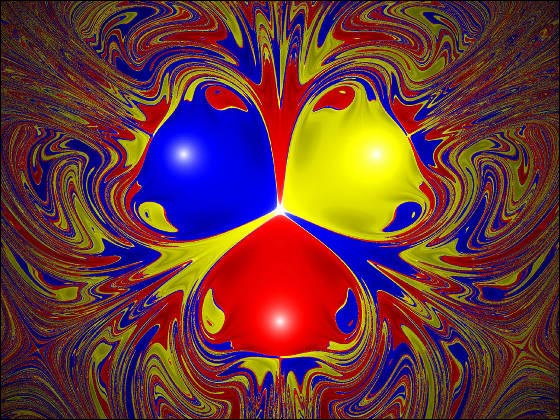20: Extra

The movies that prove "rough" the circumference and the area of ​​the circle are as follows.

Proof Without Words: The Circle - YouTube

in Science,   Video,   Design, Posted by darkhorse_log